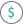#ACCRINT (PQL - xl)

This function calculates the accrued interest for a security that pays periodic interest

• Library: PQL \ Spreadsheet \ Financial
• Compatibility: Any content (regardless of data source) in the Tabulate spreadsheet module

#### Syntax

ACCRINT(issue, first interest, settlement, rate, OPTIONAL par, frequency, OPTIONAL basis)

##### Function Arguments
 Name Description Type Optional issue Date that the security is issued StringOrNumber first interest First date for calculating the interest for the security StringOrNumber settlement Settlement date for the security StringOrNumber rate Annual interest rate for the security Number par Par value for the security; if omitted, the calculation uses a value of \$1,000 Number Y frequency Frequency of payment, number of payments per year Number basis Integer representing the basis for day count Number Y